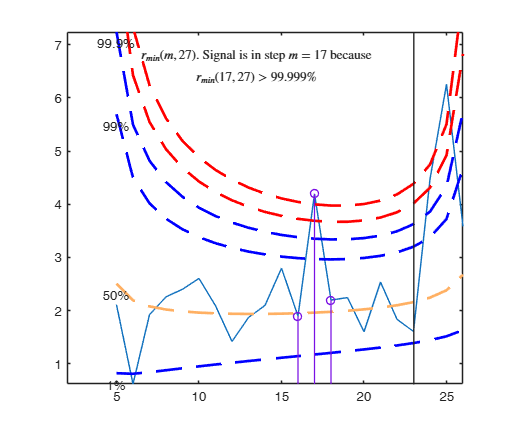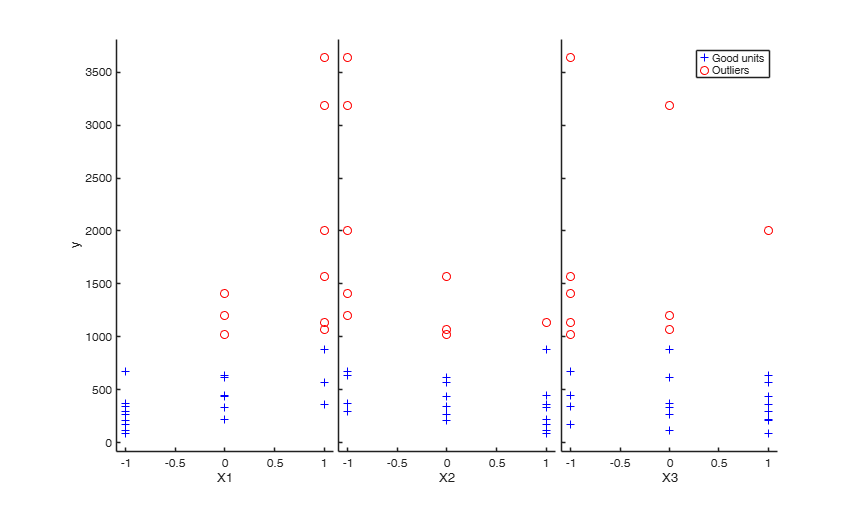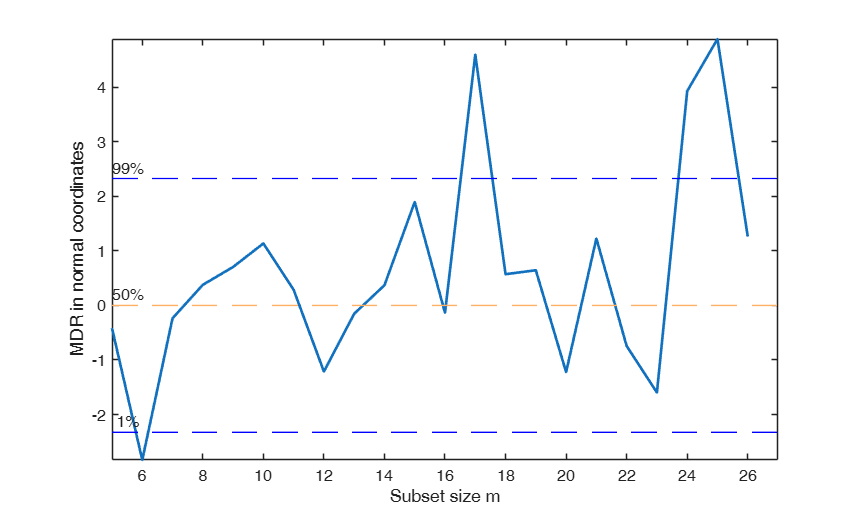# FSRinvmdr

FSRinvmdr converts values of minimum deletion residual into confidence levels

## Syntax

• MDRinv=FSRinvmdr(mdr,p)example
• MDRinv=FSRinvmdr(mdr,p,Name,Value)example

## Description

 MDRinv =FSRinvmdr(mdr, p) FSRinvmdr with all default options.

 MDRinv =FSRinvmdr(mdr, p, Name, Value) FSRinvmdr with optional arguments.

## Examples

expand all

### FSRinvmdr with all default options.

Example of finding the confidence level of MDRenv, where MDRenv is the matrix of 99 per cent confidence envelopes based on 1000 observations and 5 explanatory variables.

% MDinv is a matrix which in the second column contains
% all values equal to 0.99
p=5;
MDRenv=FSRenvmdr(1000,p,'prob',0.99);
MDRinv=FSRinvmdr(MDRenv,p);

###FSRinvmdr with optional arguments.

Example of finding confidence level of mdr for untransformed wool data.

% In the example, the values of mdr are plotted and then transformed
% into observed confidence levels.
% The output is plotted in normal coordinates.
y=wool(:,4);
X=wool(:,1:3);
% The line below shows the plot of mdr
[out]=FSR(y,X,'nsamp',0,'plots',1);
MDRinv=FSRinvmdr(out.mdr,size(X,2)+1,'plots',1);
------------------------------
Warning: Number of subsets without full rank equal to 16.3%
-------------------------
Signal detection loop
Tentative signal in central part of the search: step m=17 because
rmin(17,27)>99.999%

-------------------
Signal validation exceedance of upper envelopes
Validated signal
-------------------------------
Start resuperimposing envelopes from step m=16
Superimposition stopped because r_{min}(17,19)>99% envelope
$r_{min}(17,19)>99$\% envelope
Subsample of 18 units is homogeneous
----------------------------
Final output
Number of units declared as outliers=9
Summary of the exceedances
1          99         999        9999       99999
1           3           3           3           2## Related Examples

expand all

### Resuperimposing envelopes.

Comparison of resuperimposing envelopes using mdr coordinates and normal coordinates again on wool data.

load('wool.txt','wool');
y=wool(:,4);
X=wool(:,1:3);
% The line below shows the plot of mdr
[out]=FSR(y,X,'nsamp',0,'plots',2);
n0=16:19;
quantplo=[0.01 0.5 0.99 0.999 0.9999 0.99999];
ninv=norminv(quantplo);
lwdenv=2;
ij=0;
supn0=max(n0);
for jn0=n0;
ij=ij+1;
MDRinv = FSRinvmdr(out.mdr,4,'n',jn0);
% Resuperimposed envelope in normal coordinates
subplot(2,2,ij)
plot(MDRinv(:,1),norminv(MDRinv(:,2)),'LineWidth',2)
xlim([0 supn0])
v=axis;
line(v(1:2)',[ninv;ninv],'color','g','LineWidth',lwdenv,'LineStyle','--','Tag','env');
text(v(1)*ones(length(quantplo),1),ninv',strcat(num2str(100*quantplo'),'%'));
% line(MDRinv(:,1),norminv(MDRinv(:,2)),'LineWidth',2)
title(['Resuperimposed envelope n=' num2str(jn0)]);
end

### Example with Hospital Data.

Comparison of resuperimposing envelopes using mdr coordinates and normal coordinates at particular steps.

load('hospitalFS.txt');
y=hospitalFS(:,5);
X=hospitalFS(:,1:4);
% exploratory analysis through the yXplot
out=FSR(y,X,'nsamp',20000,'plots',2,'lms',0);
n0=[54 58 62 63];
quantplo=[0.01 0.5 0.99 0.999 0.9999 0.99999];
ninv=norminv(quantplo);
lwdenv=2;
supn0=max(n0);
figure;
ij=0;
for jn0=n0;
ij=ij+1;
[MDRinv] = FSRinvmdr(out.mdr,5,'n',jn0);
% Plot for each step of the fwd search the values of mdr translated in
% Plot for each step of the fwd search the values of mdr translated in
% terms of normal quantiles
subplot(2,2,ij)
plot(MDRinv(:,1),norminv(MDRinv(:,2)),'LineWidth',2)
xlim([0 supn0])
v=axis;
line(v(1:2)',[ninv;ninv],'color','g','LineWidth',lwdenv,'LineStyle','--','Tag','env');
text(v(1)*ones(length(quantplo),1),ninv',strcat(num2str(100*quantplo'),'%'));
line(MDRinv(:,1),norminv(MDRinv(:,2)),'LineWidth',2)
title(['Resuperimposed envelope n=' num2str(jn0)]);
end

## Input Arguments

### mdr — Minimum deletion residuals. Matrix.

n-m0 x 2 matrix containing:

1st col = fwd search index;

2nd col = minimum deletion residual .

Data Types: single| double

### p — Number of explanatory variables. Scalar.

Number of explanatory variables of the underlying dataset (including the intercept if present)

Data Types: single| double

### Name-Value Pair Arguments

Specify optional comma-separated pairs of Name,Value arguments. Name is the argument name and Value is the corresponding value. Name must appear inside single quotes (' '). You can specify several name and value pair arguments in any order as  Name1,Value1,...,NameN,ValueN.

Example:  'n',10 , 'plots',1 

### n —size of the sample.scalar.

If it is not specified it is set equal to mdr(end,1)+1

Example:  'n',10 

Data Types: double

### plots —Plot on the screen.scalar | structure.

It specify whether it is necessary to plot in normal coordinates the value of mdr If plots = 1, a plot which shows the confidence level of mdr in each step is shown on the screen.

Remark. three horizontal lines associated respectively with values 0.01 0.5 and 0.99 are added to the plot If plots is a structure the user can specify the following options conflev = vector containing horizontal lines associated with confidence levels conflevlab = scalar if it is equal 1 labels associated with horizontal lines are shown on the screen xlim = minimum and maximum on the x axis ylim = minimum and maximum on the y axis LineWidth = Line width of the trajectory of mdr in normal coordinates LineStyle = Line style of the trajectory of mle of transformation parameters LineWidthEnv = Line width of the horizontal lines Tag = tag of the plot (default is pl_mdrinv) FontSize = font size of the text labels which identify the trajectories

Example:  'plots',1 

Data Types: double

## Output Arguments

### MDRinv —Confidence levels.  Matrix

Matrix with n-m0 rows (same rows of input matrix mdr) and 3 columns:

1st col = fwd search index from m0 to n-1.

2nd col = confidence level of each value of mdr.

3rd col = confidence level in normal coordinates: 50 conf level becomes norminv(0.50)=0; 99 conf level becomes norminv(0.99)=2.33.

Atkinson, A.C. and Riani, M. (2006), Distribution theory and simulations for tests of outliers in regression, "Journal of Computational and Graphical Statistics", Vol. 15, pp. 460-476.

Riani, M. and Atkinson, A.C. (2007), Fast calibrations of the forward search for testing multiple outliers in regression, "Advances in Data Analysis and Classification", Vol. 1, pp. 123-141.